Select Committee on Public Accounts Minutes of Evidence

Value at Risk: A Worked Example

INTRODUCTION

At the recent PAC hearing on the sale of part of the UK gold reserves, the PAC Chairman, David Davies, asked for a note on value at risk. The following describes the value at risk concept and provides a worked example for two different levels of gold holding within the UK net reserves.

VALUE-AT-RISK: THE CONCEPT

Linsmeier and Pearson (1996) describe the concept of value-at-risk as, "Value at risk is a single, summary statistical measure of possible portfolio losses. Specifically, value at risk is a measure of losses due to `normal' market movements. Losses greater than the value at risk are suffered only with a specified small probability. Subject to the simplifying assumptions used in its calculation, value at risk aggregates all of the risks in a portfolio into a single number suitable for use in the boardroom, reporting to regulators, or disclosure in an annual report. Once one crosses the hurdle into using a statistical measure, the concept of value at risk is straightforward to understand. It is simply a way to describe the magnitude of the likely losses on the portfolio".

Kevin Dowd in his book "Beyond value at risk" states that, "in its most literal sense, VaR refers to a particular amount of money, the maximum amount we are likely to lose over some period, at some specific confidence level".

In other words, since risk reflects uncertain outcomes, it can usefully be described in terms of probabilities. Value-at-risk uses this approach—it is an estimate, with a given confidence level (ie some statistical probability of changes in price), of the loss that might be expected in a portfolio if positions are held over some set time horizon. It answers the question "how much can I lose with x per cent probabillity over a given time horizon".

VALUE AT RISK METHODOLOGYA HYPOTHETICAL EXAMPLE

There are three methods of calculating value at risk:

(1)  Numerical value at risk—whereby the historical movements of the portfolio as a whole are used to calculate the value at risk,

(2)  Parametric value at risk—whereby the relationships (variance and covariances) between the assets held in the portfolio are estimated and the value at risk is calculated using this information, and

(3)  Monte-Carlo simulation—whereby the value at risk is derived by simulating the relationships between the assets held in the portfolio.

The numerical method is the more intuitive of the three approaches. The following outlines how the value at risk might be calculated for a hypothetical portfolio using the numerical example. We then go on to describe, using the Parametric approach (the method most commonly used in the financial markets), how the value at risk would be calculated for the net reserves.

Figure 1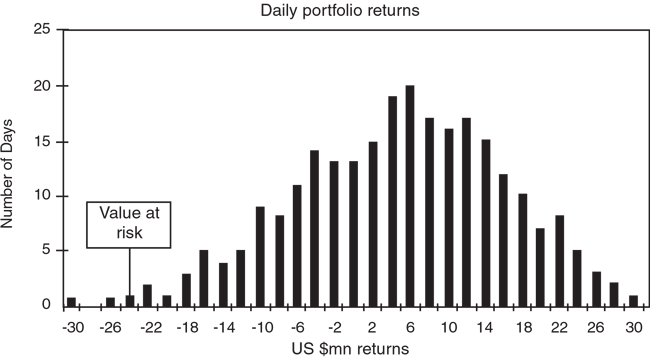Daily returns on a portfolio over time, say a year, are likely to be distributed broadly in line with those shown in figure 1. There may be a number of occasions when the portfolio made large positive returns and a number of occasions when the portfolio made large negative returns but the majority of observations will tend to lie towards the middle of the distribution, around or just above zero.

Given that we are talking about risk (Value at risk) we are only concerned with losses and, given that we are considering maximum expected losses subject to a high confidence limit, essentially with large losses, ie the observations in the left hand tail. From the hypothetical data shown in figure 1 we can see that losses of US\$30 million, US\$26 million and US\$24 million were made on one occasion each.

In determining the value at risk it is first necessary to decide on a cut off point for the confidence interval, for example 99 per cent. If we choose a 99 per cent confidence interval then we are implicitly saying that we would not expect to lose more than the value at risk figure on all but three (1 per cent of trading days) trading days per year.

Value at risk is the maximum expected loss over a given horizon period at a given level of confidence. In the above example, the maximum expected daily loss, with a 99 per cent confidence interval, is US\$24 million.

A WORKED EXAMPLE USING THE UK NET RESERVES (THE PARAMETRIC APPROACH)

Consider the case of a portfolio worth \$14 billion where gold makes up 50 per cent of the portfolio (ie \$7 billion) and the remainder is split 40:40:20 into \$, Euro and Yen (ie \$2.8 billion is held in dollars, \$2.8 billion is held in euro and \$1.4 billion is held in Yen).

If the portfolio is managed in \$, there are three currency risks—the risk arising from movements in the dollar-gold exchange rate, the risk arising from movements in the dollar-euro exchange rate and the risk arising from movements in the dollar-yen exchange rate.

The variance-covariance matrix for a ten day holding period is:
 Yen Euro Gold Yen (0.053 per cent 0.004 per cent 0.017 per cent) Euro (0.004 per cent 0.042 per cent 0.012 per cent) Gold (0.017 per cent 0.012 per cent 0.054 per cent)

(Data on variances and covariances are based on historical movements in asset prices and are published by a number of firms, for instance by JP Morgan as part of its RiskMetrics service.)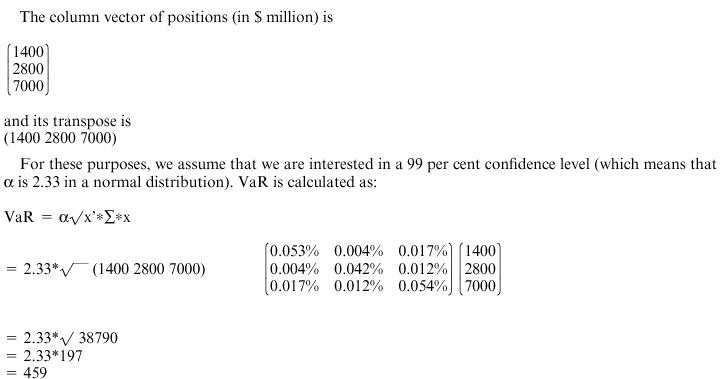So this portfolio's value-at-risk is \$459 million, ie this is the maximum amount we could expect to lose (with a 99 per cent confidence interval) over the next 10 days.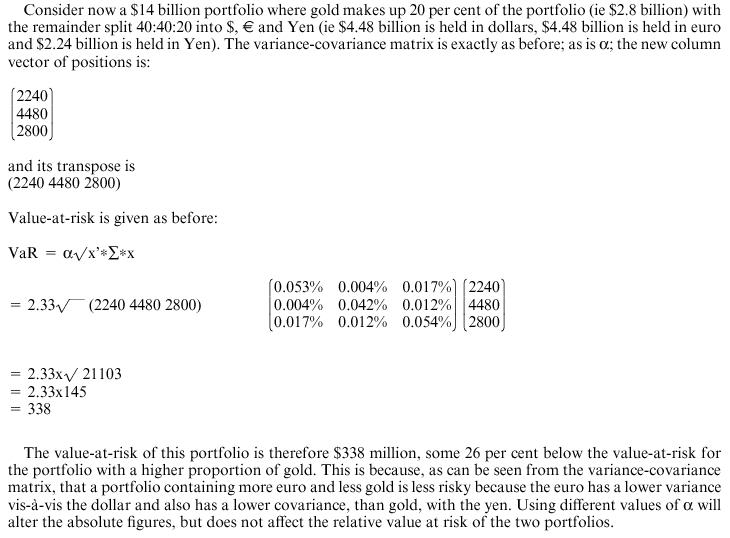CALCULATING VALUE-AT-RISK

Value-at-risk for a single position is calculated as:

VaR = sensitivity of position to change in market prices * estimated change in price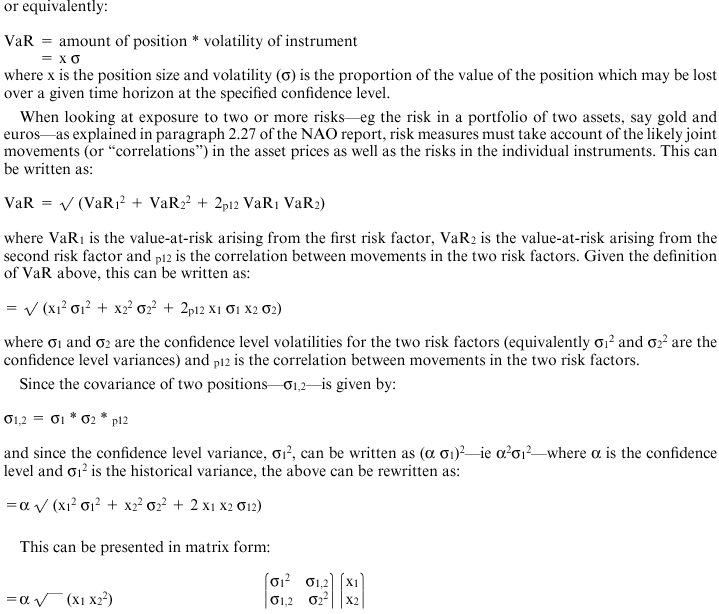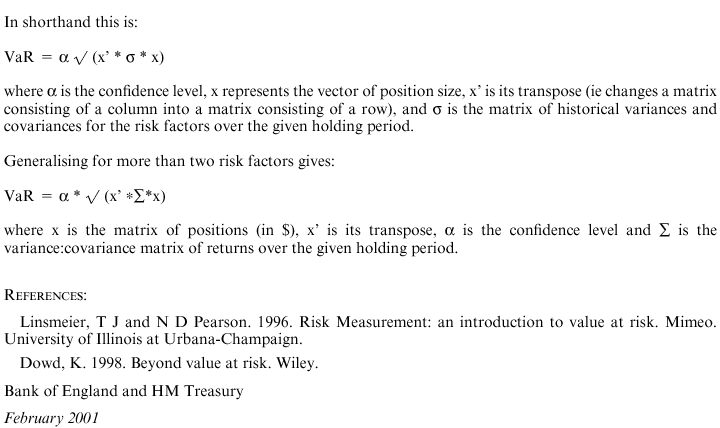8   The VaR figure, in this example, reflects the maximum amount we could expect to lose over the next 10 days. However, the variance and covariance matrix is estimated from a long run of data (eg one to two years of data). Back